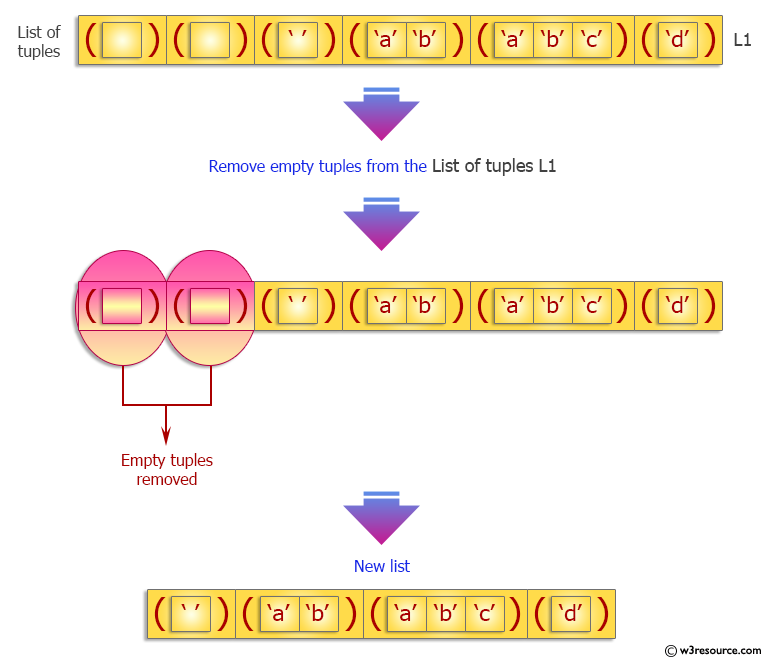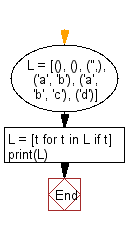﻿ Python: Remove an empty tuple(s) from a list of tuples - w3resource# Python Exercise: Remove an empty tuple(s) from a list of tuples

## Python tuple: Exercise-22 with Solution

Write a Python program to remove an empty tuple(s) from a list of tuples.

Sample Solution:-

Python Code:

``````L = [(), (), ('',), ('a', 'b'), ('a', 'b', 'c'), ('d')]
L = [t for t in L if t]
print(L)
```
```

Sample Output:

```[('',), ('a', 'b'), ('a', 'b', 'c'), 'd']
```

Pictorial Presentation:Flowchart:## Visualize Python code execution:

The following tool visualize what the computer is doing step-by-step as it executes the said program:

Python Code Editor:

Have another way to solve this solution? Contribute your code (and comments) through Disqus.

What is the difficulty level of this exercise?

Test your Programming skills with w3resource's quiz.

﻿

## Python: Tips of the Day

Decapitalizes the first letter of a string:

Example:

```def tips_decapitalize(s, upper_rest=False):
return s[:1].lower() + (s[1:].upper() if upper_rest else s[1:])
print(tips_decapitalize('PythonTips'))
print(tips_decapitalize('PythonTips', True))
```

Output:

```pythonTips
pYTHONTIPS
```